Solutions for Second Exam Review Questions        MAT 131, Fall 1998

1. Compute the derivative with respect to x for each of the following expressions: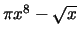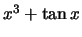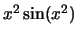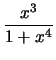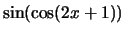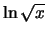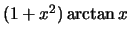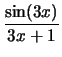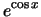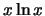Solution:

••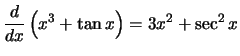•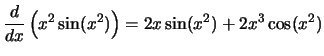•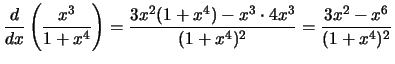•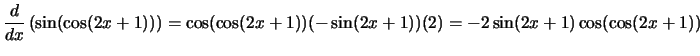•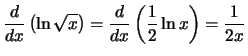•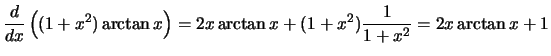•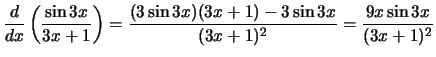•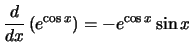•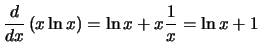2. Consider the elliptic curve C which consists of the set of points for which

x2 -x = y3 - y

(see the graph below).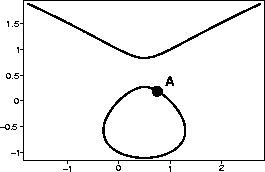a.
Write the equation of the line tangent to C at the point (1,0).

Solution:
We use implicit differentiation to obtain 2x - 1 = 3y2 y' - y'. Solving for y' gives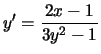. Plugging in at the point (1,0) says the slope of the relevant tangent line is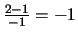. Thus, the line tangent to C at (1,0) is y = 0 -1(x -1)-- that is, y=1-x.

b.
Use your answer to part a to estimate the y-coordinate of the point with x-coordinate 3/4 marked A in the figure. Plug your estimate into the equation for C to determine how good it is.

Solution:
Plugging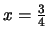into the equation for the tangent line gives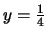. Trying the pointin the equation for C, we obtain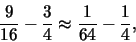which is off from being true by 3/64, or about 0.0469.

c.
Write the equation of the parabola which best approximates C at the point (1,0).

Solution:
We need to determine y'', so we take the derivative of y' from part a. Again, we use implicit differentiation, this time together with the quotient rule.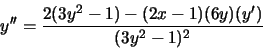Thus,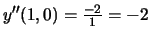. This means our desired parabola is 1 - x - (x-1)2.

d.
Use your answer to part c to improve your answer from part b. How close does this new estimate come to being right?

Solution:
Here we obtain the estimate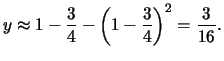Plugging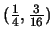into the equation for C givesoff by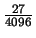, or about .00659, a dramatic improvement.

3. A mold culture is growing on the world's largest slice of bread. The culture starts in the center of the bread, and remains approximately circular.

a.
The size of the culture grows at a rate proportional to the square of its diameter. Write a differential equation which expresses this relationship.

Solution:
Let's let y(t) be the diameter of the culture. This changes at a rate proportional to (that is, a constant times) its square, so we have

y' = ky2

b.
Verify that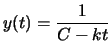satisfies the differential equation for any choice of k and C.

Solution:
We just need to check that this particular y(t) satisfies the equation in a. Taking the derivative, we have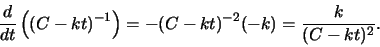This is exactly k (y(t))2, so the equation holds.

c.
If the diameter of the culture was 1 mm at 8 A.M. and 2 mm at noon, what is the size of the culture at 2 P.M.? What about at 3 P.M.? Does anything surprising happen at 4 P.M.?

Solution:
Let's let t=0 correspond to 8 A.M., so we have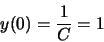That is, C=1.

Since the diameter is 2 at noon, four hours later, we have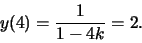Solving for k gives 1 - 4k = 1/2, or k=1/8, so our particular solution is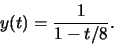At 2 P.M., the size of the culture is given by y(6) = 1/(1 - 6/8) = 4, so it is 4 mm across.

At 3 P.M., the culture has a diameter of 1/(1-7/8), doubling to 8mm in one hour.

At 4 P.M., the universe comes to an end, because the size of the mold is now infinite.

4. A spotlight is aimed at a building whose base is 20 feet away. If the light is raised so that its angle increases at a constant rate of 5 degrees per second, how fast is the image rising when the light makes a 45 degree angle with the ground?

Solution:It is helpful to draw a figure. Let's call the angle the spotlight makes with the ground A, so we have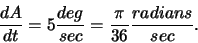Let's also call the distance from the ground to where the spotlight hits the building h, so what we want to know is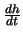when A=45 degrees, or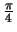radians.

It is probably safe to assume that the building is at least approximately perpendicular to the ground, so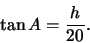Differentiating this with respect to t gives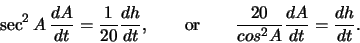Plugging in what we know gives the result: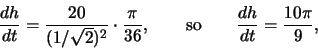or about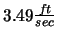.

5. A poster is to be made which requires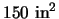for the printed part, and is to have a 3" margin at the top and bottom, and a 2" margin on the sides. What should the dimensions be in order to minimize the total area of the poster?

Solution: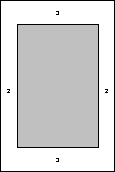If we let w represent the width of the printed message and h be its height, then hw = 150, or h = 150/w. The dimensions of the poster are 4+w wide by 6+h tall, so we want to minimize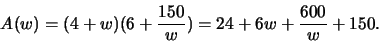Thus,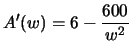, and A'(w) = 0 when w=10. So the dimensions of the optimal poster are, including the margins. The area for the message is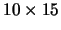.

6. The stiffness of a beam is directly proportional to the product of its width and the cube of its breadth. What are the dimensions of the stiffest beam that can be cut from a cylindrical log with a radius of 2'?

Solution: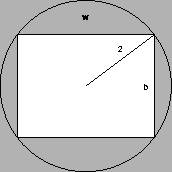We want to maximize the product w b3, where, that is,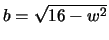. We also must have 0 < w < 4.

So,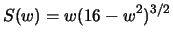, and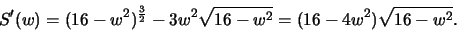S' is zero when w=4 and w=2. For w=4 we have b=0, which gives a strength of 0, as does w=0. When w=2, we have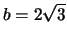, so the optimal dimensions are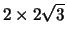.

7. At what x value does the maximum of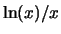occur? What is the maximum value of the function?

Solution:
Write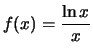. Then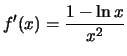. Thus, f'(x)=0 if, and f'(x) does not exist if x=0. Thus x=e is a relative maximum, and it is a global maximum because of the shape of the graph. The maximum value is 1/e.

8. Compute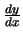for the curve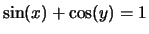. What is the slope of the tangent line at the point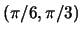?

Solution:
Using implicit differentiation,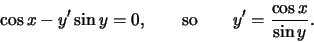At, the slope is 1.

Scott Sutherland
1998-11-11